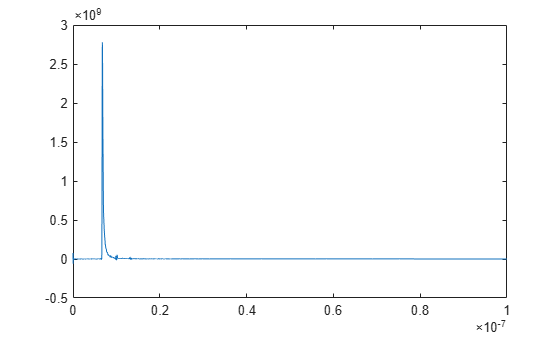# SParameterChannel

Convert S-parameter to impulse response

Since R2021a

## Description

The `SParameterChannel` object combines the scattering parameter (S-parameter) model of a baseband communication channel with an analog transmitter and receiver into a single S-parameter with adjustable port ordering. The object then calculates the impulse response of the S-Parameter using the `rational` (RF Toolbox) function. The `SParameterChannel` object supports .sNp Touchstone files, where N represents the number of ports.

## Creation

### Syntax

``obj = SParameterChannel(filename)``

### Description

````obj = SParameterChannel(filename)` creates an S-parameter channel object `obj` by importing data from the Touchstone file specified by `filename`.```

### Input Arguments

expand all

Touchstone 1.0 data file that contains network parameter data, specified as a character vector or string scalar. `filename` can be the name of a file on the MATLAB® path or the full path to a file.

Note

The data file must be formatted as a Touchstone 1.0 file. Touchstone 2.0 is not supported.

## Properties

expand all

The name of the Touchstone data file from where you want to import the S-parameter data, specified as a character vector or string scalar.

Data Types: `char` | `string`

Port order for the S-parameter, specified as a character vector. If left blank, the function automatically detects the through paths and the re-orders the ports in the order [in1 out1 in2 out2].

Example: [1 2 3 4]

Data Types: `char`

Maximum number of poles to use to fit a rational model, specified as a positive scalar integer.

Data Types: `double`

Desired error tolerance for rational model fit, specified as a real scalar in dB.

Data Types: `double`

Sample interval of the impulse response, specified as a positive real scalar in seconds.

Data Types: `double`

Desired duration of the impulse response, specified as a positive real scalar in seconds. The total number of samples in the impulse response is calculated by dividing the `StopTime` property by `SampleInterval` property.

Data Types: `double`

Single-ended impedance of the transmitter analog model, specified as a nonnegative real scalar in ohms.

Data Types: `double`

Capacitance of the transmitter analog model, specified as a nonnegative real scalar in farads.

Data Types: `double`

Amplitude of the stimulus input to the transmitter analog model, specified as a real scalar.

Data Types: `double`

20%–80% rise time of the stimulus input to the transmitter analog model, specified as a positive real scalar in seconds.

Data Types: `double`

Single-ended impedance of the receiver analog model, specified as a nonnegative real scalar in ohms.

Data Types: `double`

Single-ended impedance of the receiver analog model, specified as a nonnegative real scalar in ohms.

Data Types: `double`

Port order used for the S-parameter channel analysis, returned as a vector. If you do not define the port order using `PortOrder` property, the through paths are automatically detected and reported here.

Data Types: `double`

Impulse response of the system defined by the S-parameter channel object, returned as a vector.

Data Types: `double`

Time vector corresponding to the system impulse responses, returned as a vector.

Data Types: `double`

S-parameter frequencies, specified as a positive real-valued vector. The frequencies are sorted from the smallest to the largest value.

Data Types: `double`

Transfer function of the system defined by the S-parameter channel object, returned as a vector.

Data Types: `double`

Output statistics from `rational` (RF Toolbox), returned as an object.

Data Types: `double`

Uncertainty due to rise time discretization, returned as a negative real scalar.

Data Types: `double`

## Examples

collapse all

Create an S-parameter channel object.

`obj = SParameterChannel('FileName','default.s4p');`

Extract the time vectors and impulse responses.

```t = obj.t; impulse = obj.ImpulseResponse;```

Plot the impulse response.

`plot(t,impulse);`## Version History

Introduced in R2021a